# Calculate the current (in Amps) passing through each resistor shown in the screen shot of a...

Calculate the current (in Amps) passing through each resistor shown in the screen shot of a virtual circuit and thus prove the measurements in the ammeters next to each resistor. Please use ALL THREE of Kirchhoff’s Rules and show work. Thank you.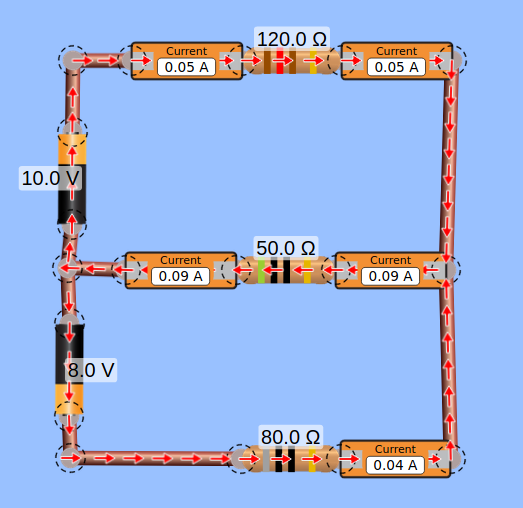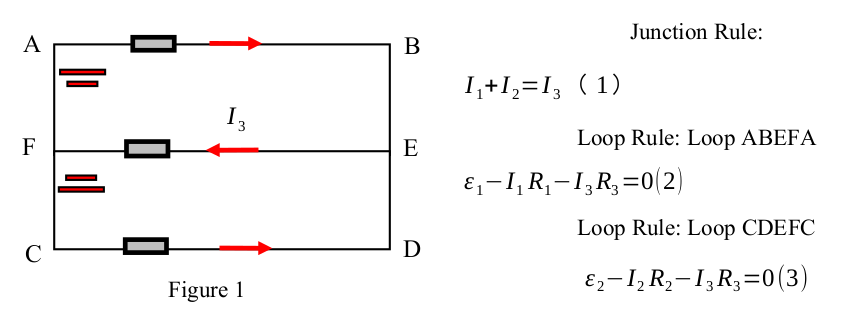120.02 Current 0.05 A Current 0.05 A 10.0 v 50.02 Current 0.09 A Current 0.09 A 8.0 V 80.0 12 Current 0.04 A
Junction Rule: A B 11+12=12 (1) 13 3 F E Loop Rule: Loop ABEFA &1-1,R2-13R3 =0(2) Loop Rule: Loop CDEFC C D Figure 1 €2-1, R2–13 Rz=0(3)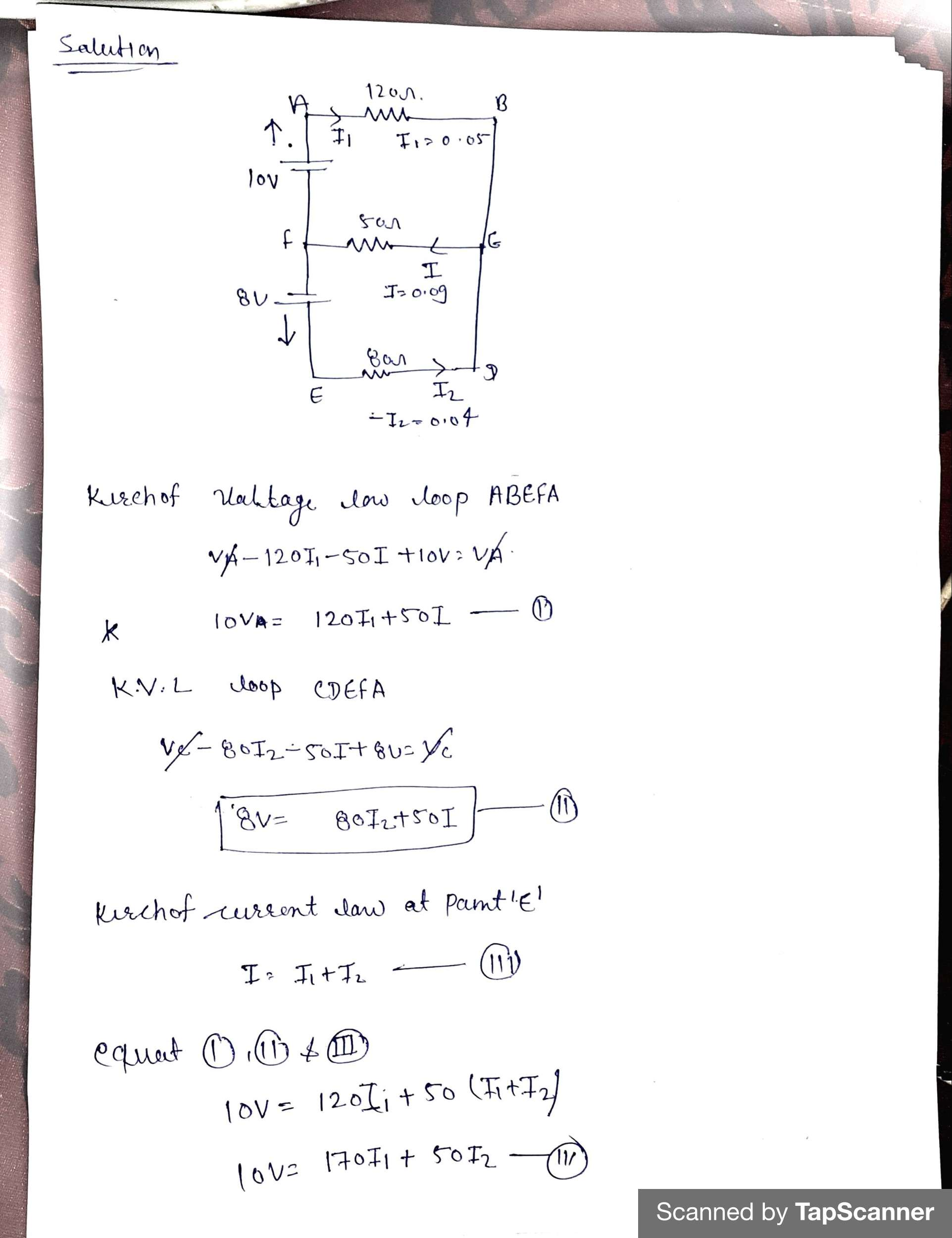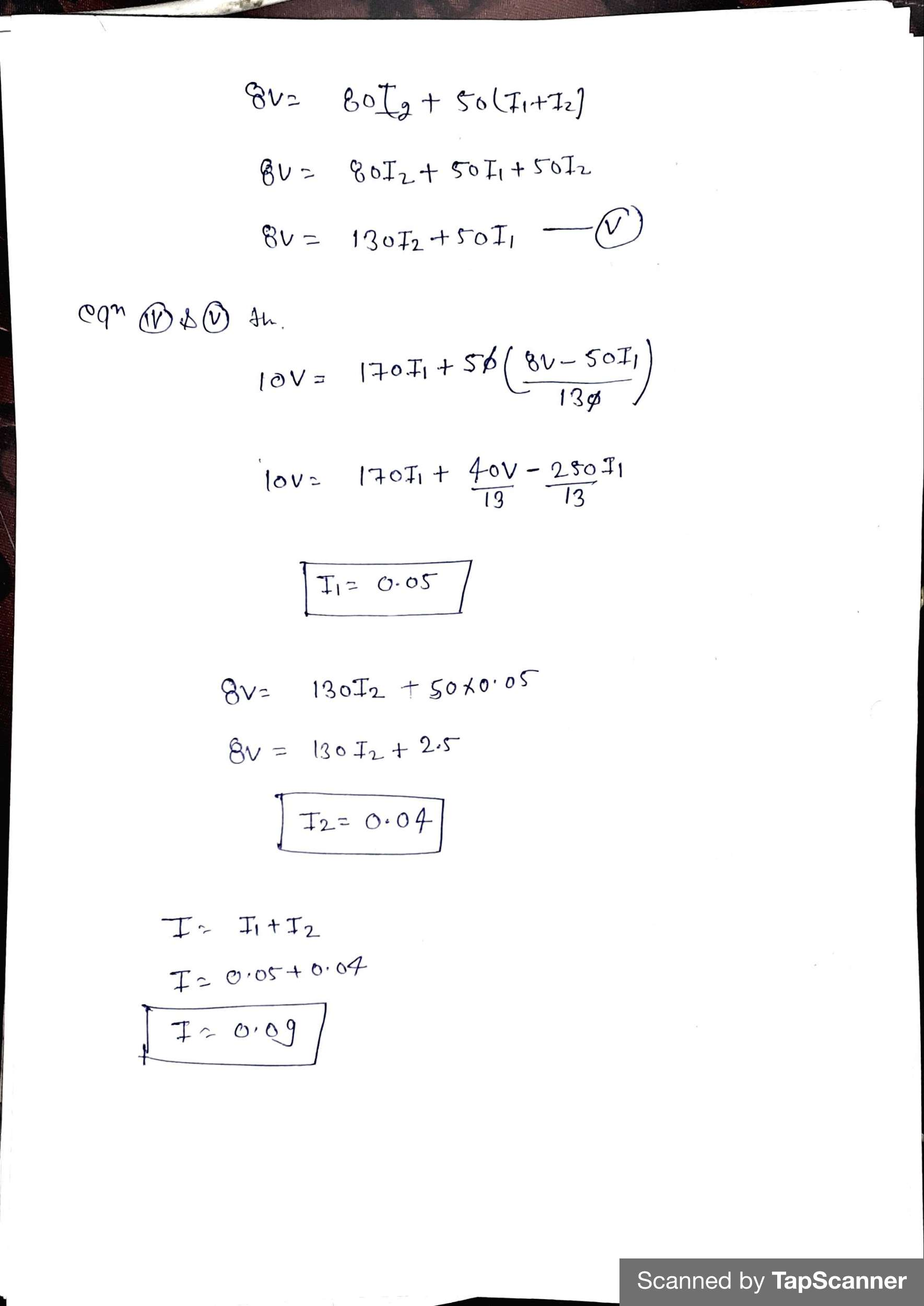##### Add Answer of: Calculate the current (in Amps) passing through each resistor shown in the screen shot of a...
Similar Homework Help Questions
• ### Junction Rule: I1+I2=I3                     （1） Loop Rule: Loop ABEFA ε1-I1R1-I3R3=0          2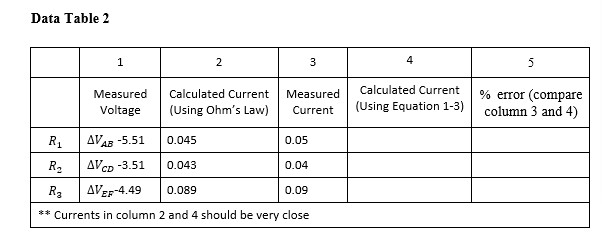Junction Rule: I1+I2=I3                     （1） Loop Rule: Loop ABEFA ε1-I1R1-I3R3=0          2 Loop Rule: Loop CDEFC ε2-I2R2-I3R3=0          (3) HOW DO YOU FIND THE CALCULATED CURRENT PLEASE SHOW THE STEPS! THANK YOU Data Table 2 1 2 3 4 5 Measured Voltage Calculated Current (Using Ohm's Law) Measured Current Calculated Current (Using Equation 1-3) % error (compare column 3 and 4) R1 AVAB -5.51 0.045 0.05 R2 AVCD -3.51 0.043 0.04 RE AVEF-4.49 0.089 0.09 ** Currents in column 2 and 4 should...

• ### please show formulas used and round four significant figures. thanks Find the current passing through each...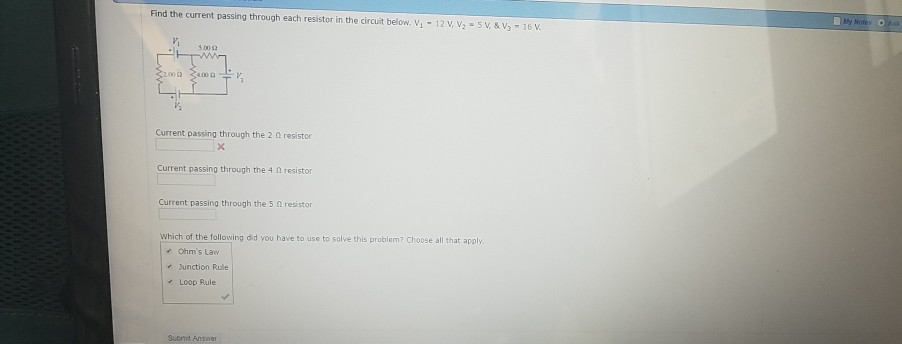please show formulas used and round four significant figures. thanks Find the current passing through each resistor in the circuit below. V, - 12 V, V = 5V & V, - 16 V. 5000 ch 31000 3100 Current passing through the 20 resistor Current passing through the 4 n resistor Current passing through the 5 resistor Which of the following did you have to use to solve this problem? Choose all that apply Ohm's Law Junction Rule Loop Rule Submit...

• ### Q.2: Using the loop rule, find the current flowing through each resistor. Given Rı=48.00, R2=8.092, Rz=16.022...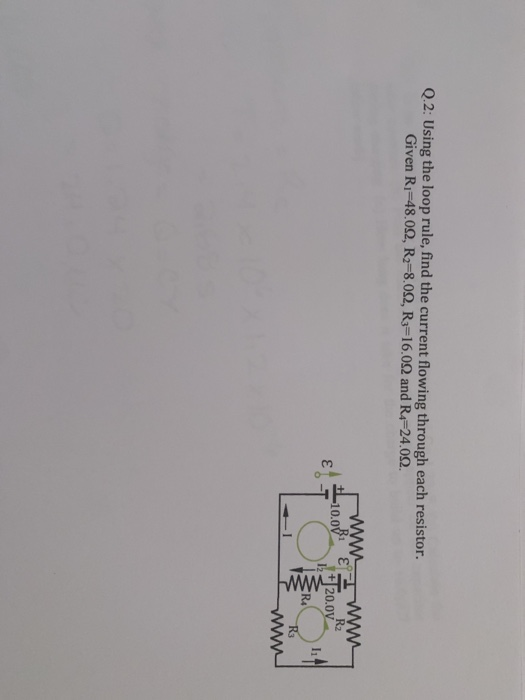Q.2: Using the loop rule, find the current flowing through each resistor. Given Rı=48.00, R2=8.092, Rz=16.022 and R=24.09. +L10.00 120.0V R3 L'em

• ### How do I calculate the “calculated current flowing through each resistor using Kirchhoffs junction rule and...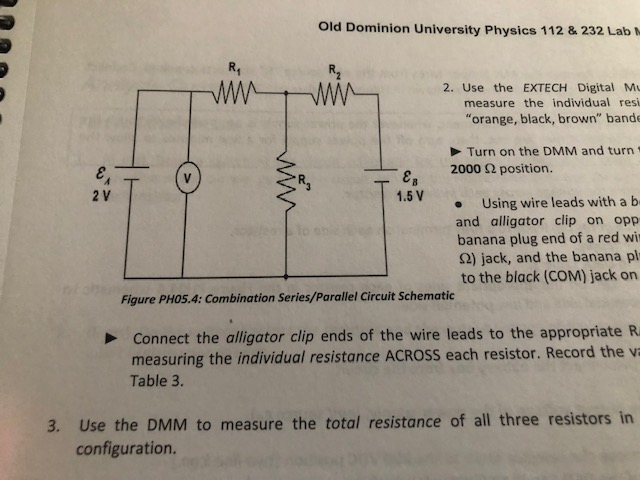How do I calculate the “calculated current flowing through each resistor using Kirchhoffs junction rule and the measured values”? Please show your work. Thank you! Old Dominion University Physics 112 & 232 Lab 2. Use the EXTECH Digital M measure the individual resi "orange, black, brown" band Turn on the DMM and turn 2000 Ω position. 2 V 1.5 V Using wire leads with ab and alligator clip on opp banana plug end of a red wi 2) jack, and...

• ### For the circuit below, determine the MAGNITUDE of the CURRENT passing through each resistor. V1 =...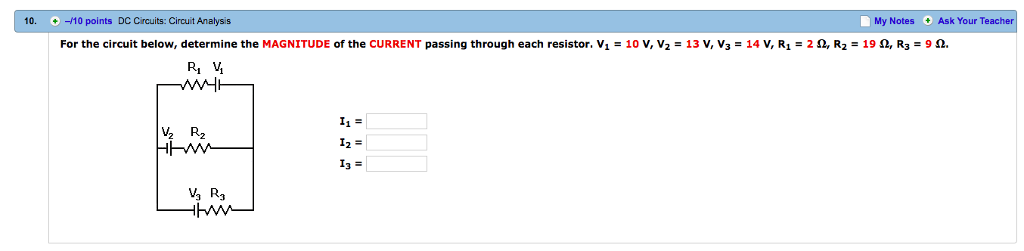For the circuit below, determine the MAGNITUDE of the CURRENT passing through each resistor. V1 = 10 V, V2 = 13 V, V3 = 14 V, R1 = 2 Ω, R2 = 19 Ω, R3 = 9 Ω. Please show all work. 10. -10 points DC Circuits: Circuit Analysis My Notes Ask Your For the circuit below, determine the MAGNITUDE of the CURRENT passing through each resistor. V1 = 10 V, V2 13 V, V3 = 14 V, R1 =...

• ### 1. Calculate the equivalent resistance, current through the battery, current through each resistor, and voltage across...

1. Calculate the equivalent resistance, current through the battery, current through each resistor, and voltage across each resistor for the following circuit: 1.0Ω R 2 R3 2 2.0 8.0 Ω 13.0V Ibatr I (12)- 1 (2Ω) 1 (80) V (12) V (20) V (80) =

• ### 7) You will eventually be asked to determine the current in each branch of the circuit....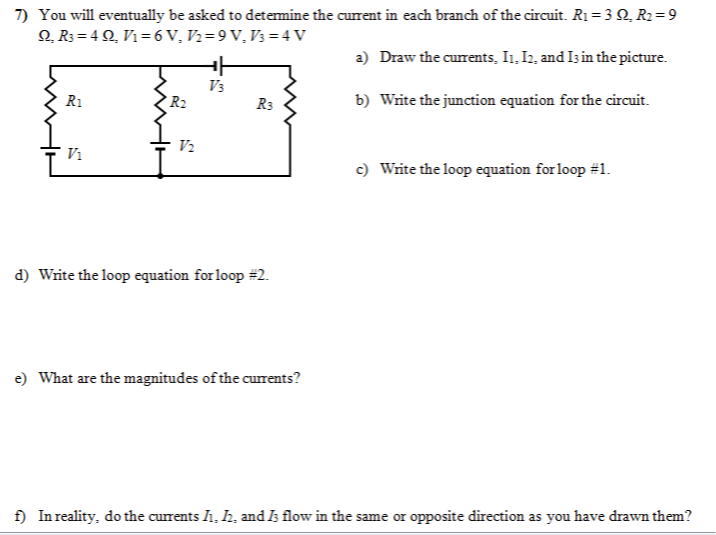7) You will eventually be asked to determine the current in each branch of the circuit. Ri=30. R2=9 2. R3 = 4 . Vi=6 V. 72=9V.V3=4 V a) Draw the currents. I1. 12. and I3 in the picture. V3 Ri R2 R3 b) Write the junction equation for the circuit. + Vi 12 c) Write the loop equation for loop #1. d) Write the loop equation for loop =2. e) What are the magnitudes of the currents? f) In reality,...

• ### Question 3. (10 points) Four resistors are connected as shown in Figure 28.9a Find the equivalent resistance between a and c. R1-16 and R4-12 Ω R2-13 R3-4 (a) (b) What is the current in each resistor...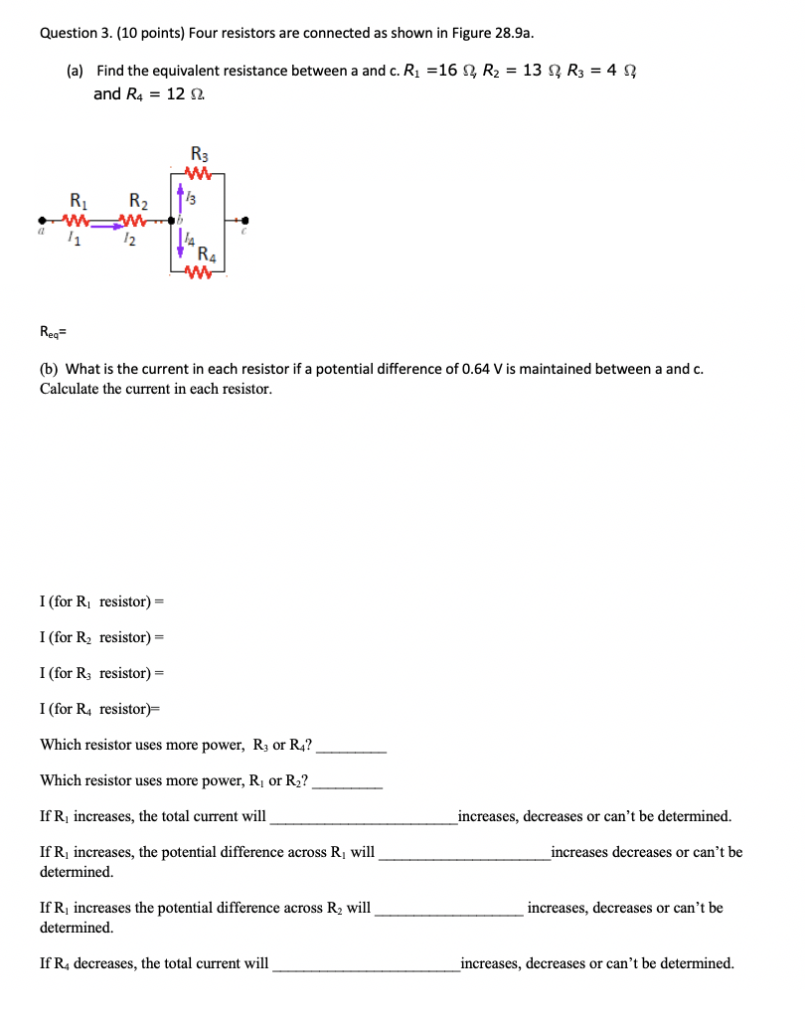Question 3. (10 points) Four resistors are connected as shown in Figure 28.9a Find the equivalent resistance between a and c. R1-16 and R4-12 Ω R2-13 R3-4 (a) (b) What is the current in each resistor if a potential difference of 0.64 V is maintained between a and c. Calculate the current in each resistor I (for Ri resistor)- I (for R2 resistor)- I (for R3 resistor) I (for R4 resistor)- Which resistor uses more power, R or R4? Which...

• ### ri R\$ 7:) Here, each V represents a change in voltage (in volts) at a battery,...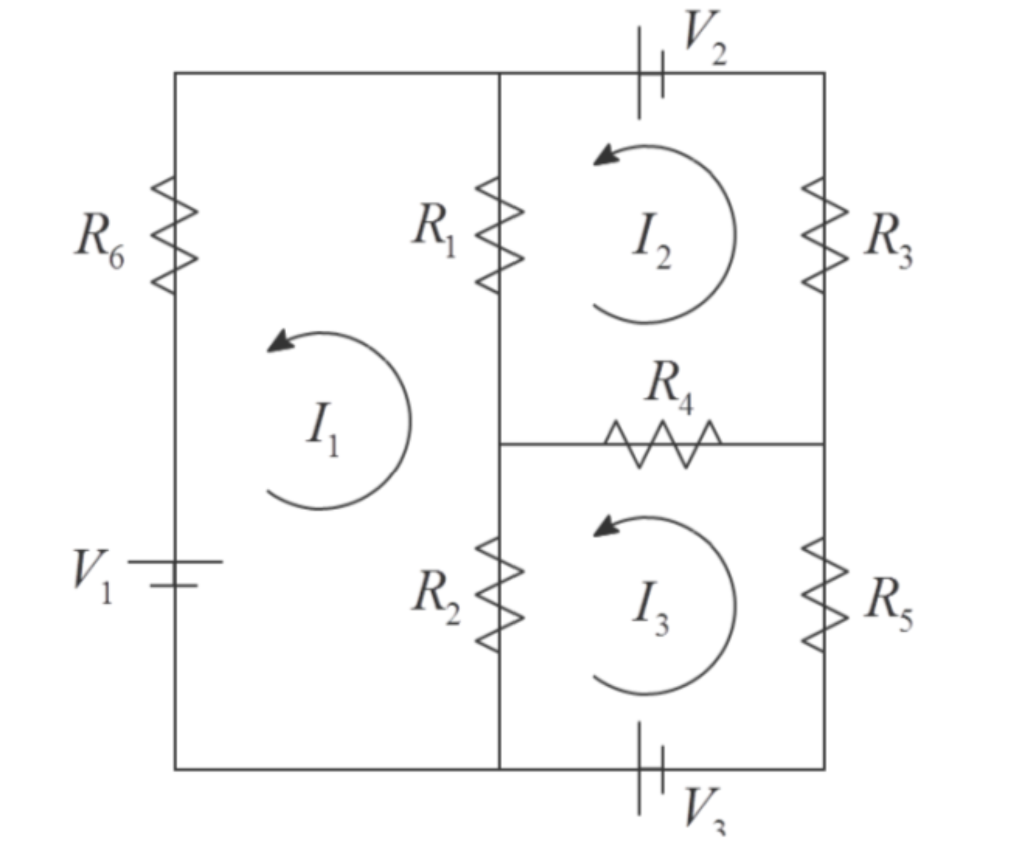ri R\$ 7:) Here, each V represents a change in voltage (in volts) at a battery, each R represents a resistance (in ohms) at a resistor and each I represents a current (in amps) through a wire. These quantities obey two simple laws: 1. Ohm's law: The voltage drop across a resistor is V = IR. 2. Kirchhoff's second law: The sum of all the voltage changes in a closed loop is zero. Using these two laws, we can construct...

• ### +Vs- -V 8 LBC BLS 11 Als 12 11 E Loop (1) R2 Loop (2)R3 L2...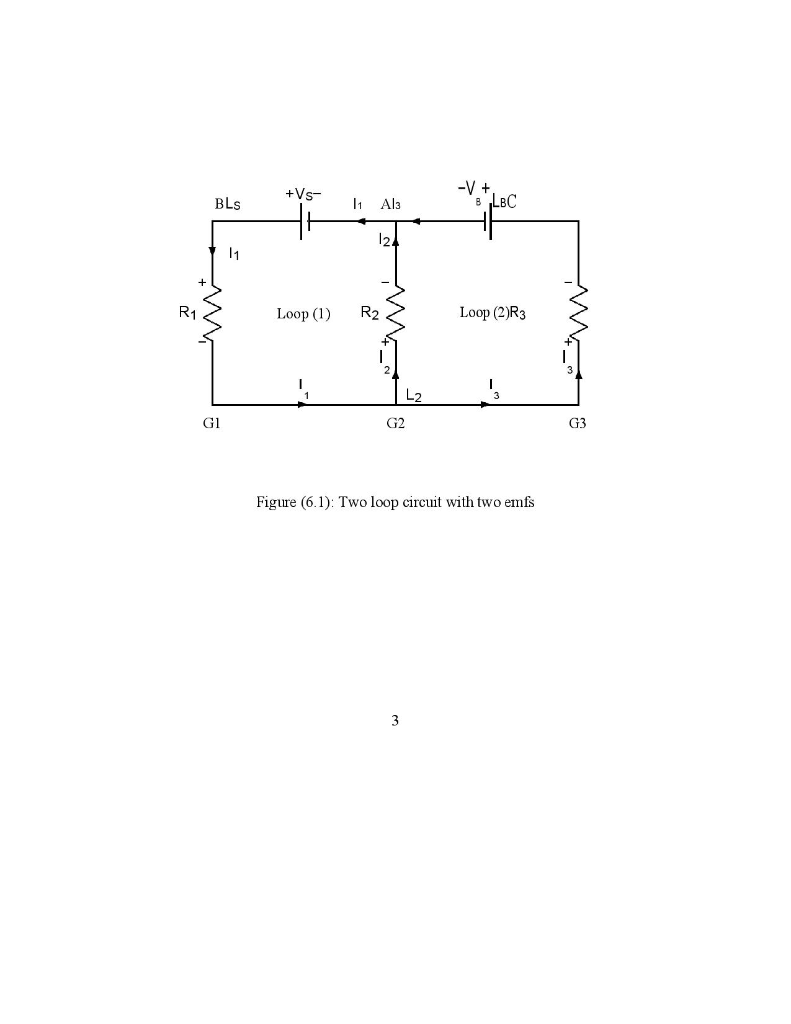+Vs- -V 8 LBC BLS 11 Als 12 11 E Loop (1) R2 Loop (2)R3 L2 GI G2 G3 Figure (6.1): Two loop circuit with two emfs 3 B A R1 R2 R3Z GI G2 G3 Figure (6.2): Series and parallel resistors + Vs - B 11 A 13 С 12 11 R1 Red R3 GI G2 G3 Figure (6.3): Series and parallel resistor circuit 4 V. ANALYSIS: Procedure (1): Two Loop Circuit 1. Verify KLR for each loop by...

Free Homework App